# Todays topics Boolean algebra preview Propositional equivalences Predicate

• Slides: 52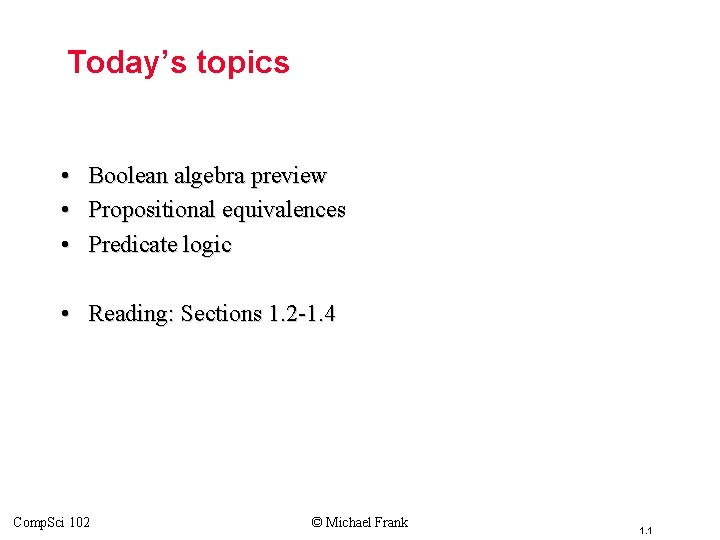Today’s topics • • • Boolean algebra preview Propositional equivalences Predicate logic • Reading: Sections 1. 2 -1. 4 Comp. Sci 102 © Michael Frank 1. 1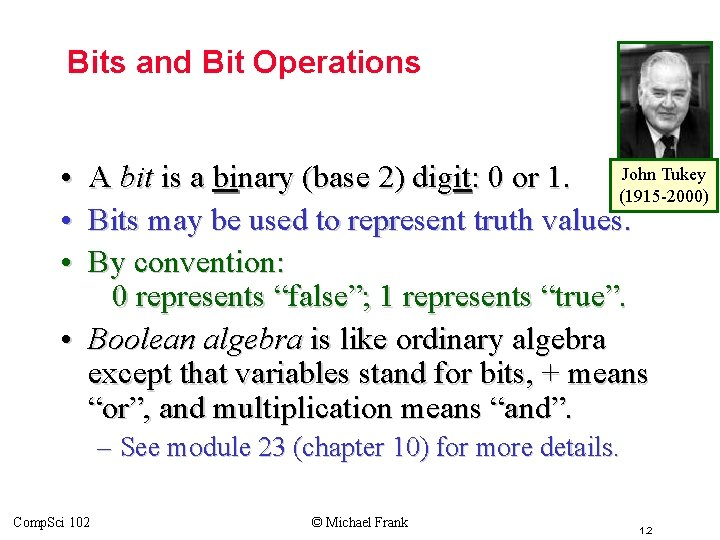Topic #2 – Bits and Bit Operations • • • John Tukey A bit is a binary (base 2) digit: 0 or 1. (1915 -2000) Bits may be used to represent truth values. By convention: 0 represents “false”; 1 represents “true”. • Boolean algebra is like ordinary algebra except that variables stand for bits, + means “or”, and multiplication means “and”. – See module 23 (chapter 10) for more details. Comp. Sci 102 © Michael Frank 1. 2Topic #2 – Bits Bit Strings • A Bit string of length n is an ordered sequence (series, tuple) of n 0 bits. – More on sequences in § 3. 2. • By convention, bit strings are (sometimes) written left to right: – e. g. the “first” bit of the bit string “ 1001101010” is 1. – Watch out! Another common convention is that the rightmost bit is bit #0, the 2 nd-rightmost is bit #1, etc. • When a bit string represents a base-2 number, by convention, the first (leftmost) bit is the most significant bit. Ex. 11012=8+4+1=13. Comp. Sci 102 © Michael Frank 1. 3Topic #2 – Bits Counting in Binary • Did you know that you can count to 1, 023 just using two hands? – How? Count in binary! • Each finger (up/down) represents 1 bit. • To increment: Flip the rightmost (low-order) bit. – If it changes 1→ 0, then also flip the next bit to the left, • If that bit changes 1→ 0, then flip the next one, etc. • 00000, 000001, 000010, … …, 111101, 111110, 11111 Comp. Sci 102 © Michael Frank 1. 4Topic #2 – Bits Bitwise Operations • Boolean operations can be extended to operate on bit strings as well as single bits. • E. g. : 01 1011 0110 11 0001 11 1011 1111 Bit-wise OR 01 0001 0100 Bit-wise AND 10 1011 Bit-wise XOR Comp. Sci 102 © Michael Frank 1. 5End of § 1. 1 You have learned about: • Propositions: What they are. • Propositional logic operators’ – – Comp. Sci 102 Symbolic notations. English equivalents. Logical meaning. Truth tables. • Atomic vs. compound propositions. • Alternative notations. • Bits and bit-strings. • Next section: § 1. 2 © Michael Frank – Propositional equivalences. – How to prove them. 1. 6Topic #1. 1 – Propositional Logic: Equivalences Propositional Equivalence (§ 1. 2) Two syntactically (i. e. , textually) different compound propositions may be the semantically identical (i. e. , have the same meaning). We call them equivalent. Learn: • Various equivalence rules or laws. • How to prove equivalences using symbolic derivations. Comp. Sci 102 © Michael Frank 1. 7Topic #1. 1 – Propositional Logic: Equivalences Tautologies and Contradictions A tautology is a compound proposition that is true no matter what the truth values of its atomic propositions are! Ex. p p [What is its truth table? ] A contradiction is a compound proposition that is false no matter what! Ex. p p [Truth table? ] Other compound props. are contingencies. Comp. Sci 102 © Michael Frank 1. 8Topic #1. 1 – Propositional Logic: Equivalences Logical Equivalence Compound proposition p is logically equivalent to compound proposition q, written p q, IFF the compound proposition p q is a tautology. Compound propositions p and q are logically equivalent to each other IFF p and q contain the same truth values as each other in all rows of their truth tables. Comp. Sci 102 © Michael Frank 1. 9Topic #1. 1 – Propositional Logic: Equivalences Proving Equivalence via Truth Tables Ex. Prove that p q ( p q). F T T T Comp. Sci 102 T T T F F F © Michael Frank F T T T 1. 10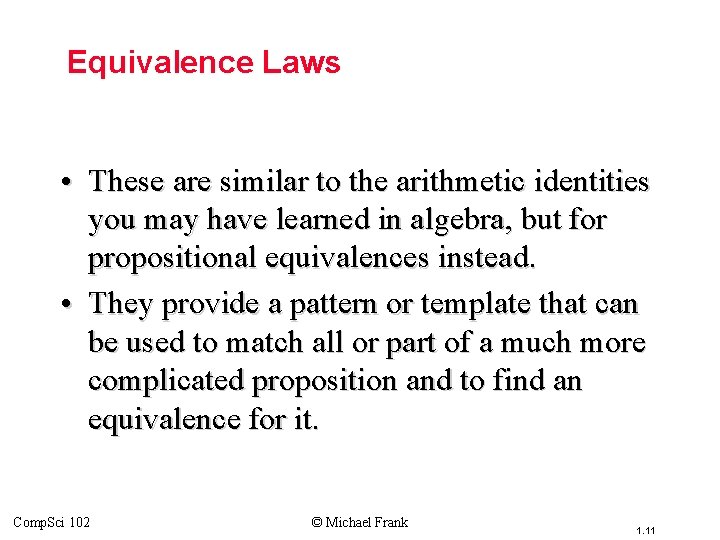Topic #1. 1 – Propositional Logic: Equivalences Equivalence Laws • These are similar to the arithmetic identities you may have learned in algebra, but for propositional equivalences instead. • They provide a pattern or template that can be used to match all or part of a much more complicated proposition and to find an equivalence for it. Comp. Sci 102 © Michael Frank 1. 11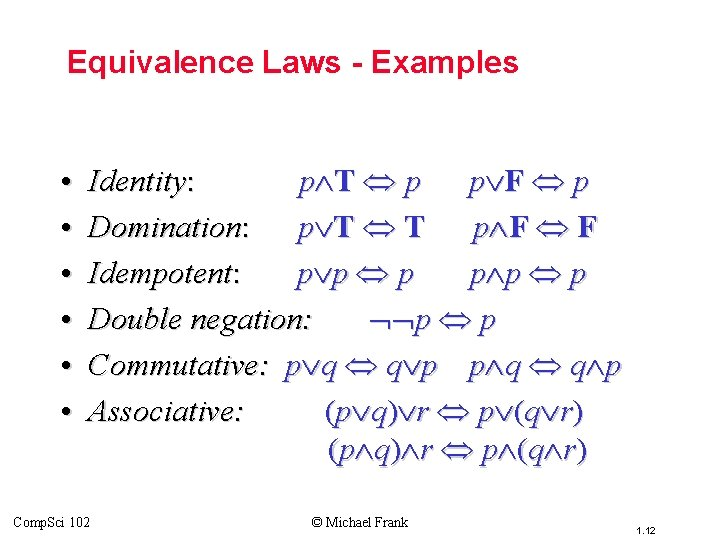Topic #1. 1 – Propositional Logic: Equivalences Equivalence Laws - Examples • • • Identity: p T p p F p Domination: p T T p F F Idempotent: p p p Double negation: p p Commutative: p q q p Associative: (p q) r p (q r) Comp. Sci 102 © Michael Frank 1. 12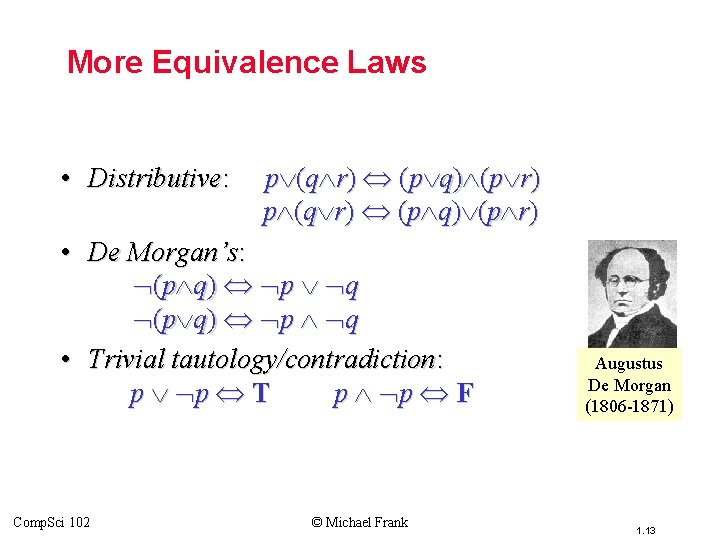Topic #1. 1 – Propositional Logic: Equivalences More Equivalence Laws • Distributive: p (q r) (p q) (p r) • De Morgan’s: (p q) p q • Trivial tautology/contradiction: p p T p p F Comp. Sci 102 © Michael Frank Augustus De Morgan (1806 -1871) 1. 13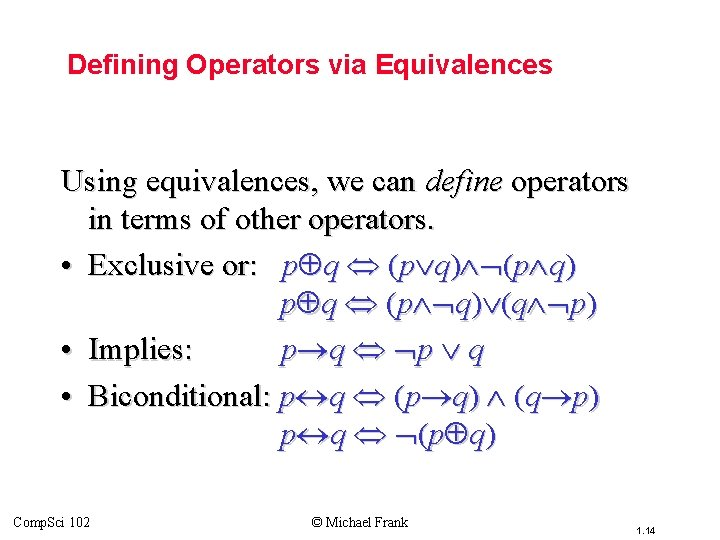Topic #1. 1 – Propositional Logic: Equivalences Defining Operators via Equivalences Using equivalences, we can define operators in terms of other operators. • Exclusive or: p q (p q) (q p) • Implies: p q p q • Biconditional: p q (p q) (q p) p q (p q) Comp. Sci 102 © Michael Frank 1. 14Topic #1. 1 – Propositional Logic: Equivalences An Example Problem • Check using a symbolic derivation whether (p q) (p r) p q r. (p q) (p r) [Expand definition of ] (p q) (p r) [Expand defn. of ] (p q) ((p r) (p r)) [De. Morgan’s Law] ( p q) ((p r) (p r)) cont. Comp. Sci 102 © Michael Frank 1. 15Topic #1. 1 – Propositional Logic: Equivalences Example Continued. . . ( p q) ((p r) (p r)) [ commutes] (q p) ((p r) (p r)) [ associative] q ( p ((p r) (p r))) [distrib. over ] q ((( p (p r)) ( p (p r))) [assoc. ] q ((( p p) r) ( p (p r))) [trivail taut. ] q ((T r) ( p (p r))) [domination] q (T ( p (p r))) [identity] q ( p (p r)) cont. Comp. Sci 102 © Michael Frank 1. 16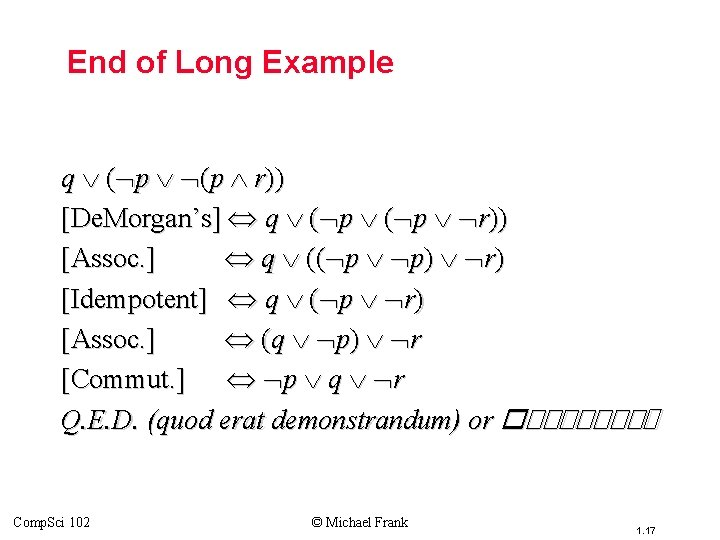Topic #1. 1 – Propositional Logic: Equivalences End of Long Example q ( p (p r)) [De. Morgan’s] q ( p r)) [Assoc. ] q (( p p) r) [Idempotent] q ( p r) [Assoc. ] (q p) r [Commut. ] p q r Q. E. D. (quod erat demonstrandum) or ������� Comp. Sci 102 © Michael Frank 1. 17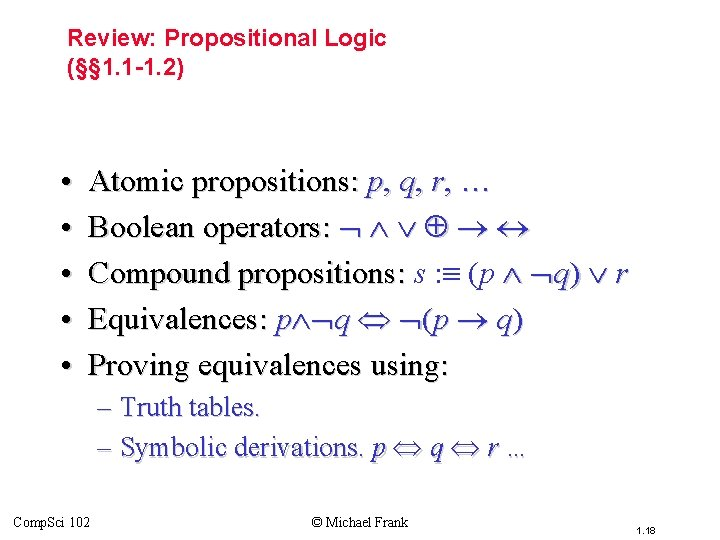Review: Propositional Logic (§§ 1. 1 -1. 2) • • • Topic #1 – Propositional Logic Atomic propositions: p, q, r, … Boolean operators: Compound propositions: s : (p q) r Equivalences: p q (p q) Proving equivalences using: – Truth tables. – Symbolic derivations. p q r … Comp. Sci 102 © Michael Frank 1. 18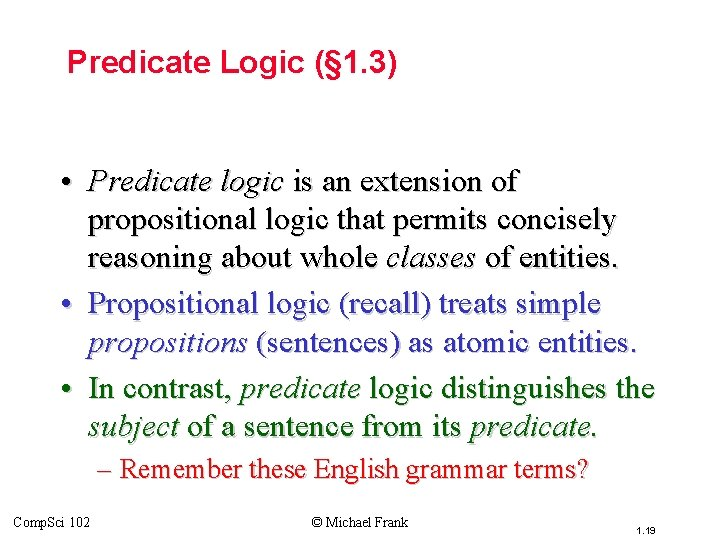Topic #3 – Predicate Logic (§ 1. 3) • Predicate logic is an extension of propositional logic that permits concisely reasoning about whole classes of entities. • Propositional logic (recall) treats simple propositions (sentences) as atomic entities. • In contrast, predicate logic distinguishes the subject of a sentence from its predicate. – Remember these English grammar terms? Comp. Sci 102 © Michael Frank 1. 19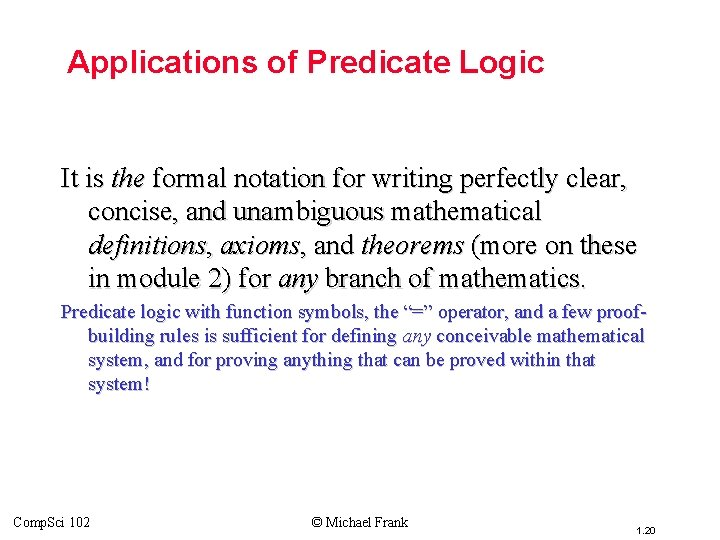Topic #3 – Predicate Logic Applications of Predicate Logic It is the formal notation for writing perfectly clear, concise, and unambiguous mathematical definitions, axioms, and theorems (more on these in module 2) for any branch of mathematics. Predicate logic with function symbols, the “=” operator, and a few proofbuilding rules is sufficient for defining any conceivable mathematical system, and for proving anything that can be proved within that system! Comp. Sci 102 © Michael Frank 1. 20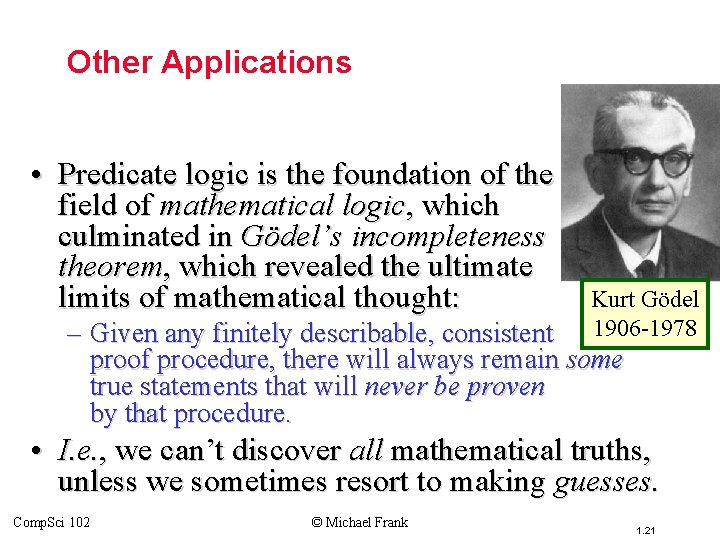Topic #3 – Predicate Logic Other Applications • Predicate logic is the foundation of the field of mathematical logic, which culminated in Gödel’s incompleteness theorem, which revealed the ultimate limits of mathematical thought: Kurt Gödel 1906 -1978 – Given any finitely describable, consistent proof procedure, there will always remain some true statements that will never be proven by that procedure. • I. e. , we can’t discover all mathematical truths, unless we sometimes resort to making guesses. Comp. Sci 102 © Michael Frank 1. 21Practical Applications of Predicate Logic Topic #3 – Predicate Logic • It is the basis for clearly expressed formal specifications for any complex system. • It is basis for automatic theorem provers and many other Artificial Intelligence systems. – E. g. automatic program verification systems. • Predicate-logic like statements are supported by some of the more sophisticated database query engines and container class libraries – these are types of programming tools. Comp. Sci 102 © Michael Frank 1. 22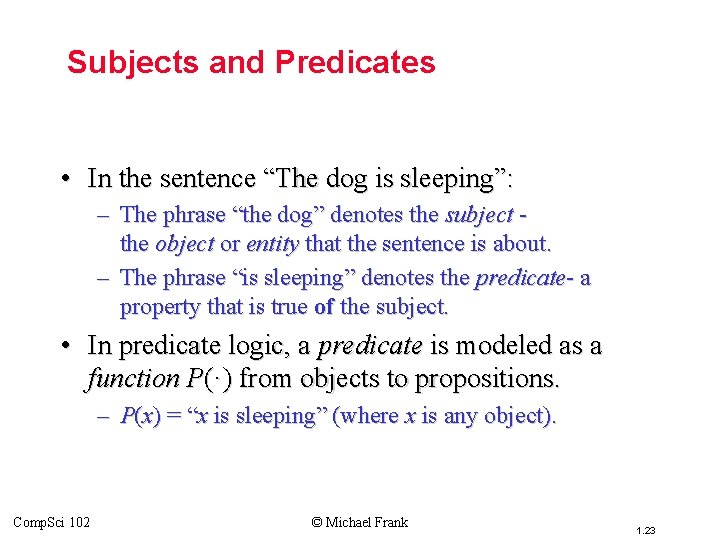Topic #3 – Predicate Logic Subjects and Predicates • In the sentence “The dog is sleeping”: – The phrase “the dog” denotes the subject the object or entity that the sentence is about. – The phrase “is sleeping” denotes the predicate- a property that is true of the subject. • In predicate logic, a predicate is modeled as a function P(·) from objects to propositions. – P(x) = “x is sleeping” (where x is any object). Comp. Sci 102 © Michael Frank 1. 23Topic #3 – Predicate Logic More About Predicates • Convention: Lowercase variables x, y, z. . . denote objects/entities; uppercase variables P, Q, R… denote propositional functions (predicates). • Keep in mind that the result of applying a predicate P to an object x is the proposition P(x). But the predicate P itself (e. g. P=“is sleeping”) is not a proposition (not a complete sentence). – E. g. if P(x) = “x is a prime number”, P(3) is the proposition “ 3 is a prime number. ” Comp. Sci 102 © Michael Frank 1. 24Topic #3 – Predicate Logic Propositional Functions • Predicate logic generalizes the grammatical notion of a predicate to also include propositional functions of any number of arguments, each of which may take any grammatical role that a noun can take. – E. g. let P(x, y, z) = “x gave y the grade z”, then if x=“Mike”, y=“Mary”, z=“A”, then P(x, y, z) = “Mike gave Mary the grade A. ” Comp. Sci 102 © Michael Frank 1. 25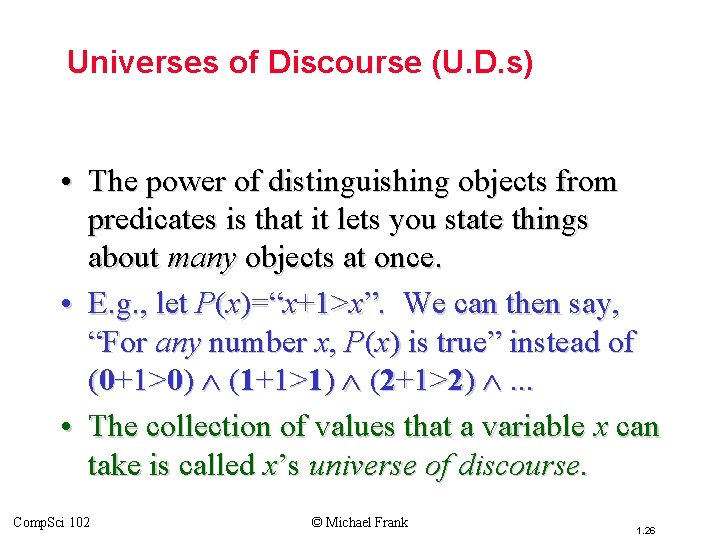Topic #3 – Predicate Logic Universes of Discourse (U. D. s) • The power of distinguishing objects from predicates is that it lets you state things about many objects at once. • E. g. , let P(x)=“x+1>x”. We can then say, “For any number x, P(x) is true” instead of (0+1>0) (1+1>1) (2+1>2) . . . • The collection of values that a variable x can take is called x’s universe of discourse. Comp. Sci 102 © Michael Frank 1. 26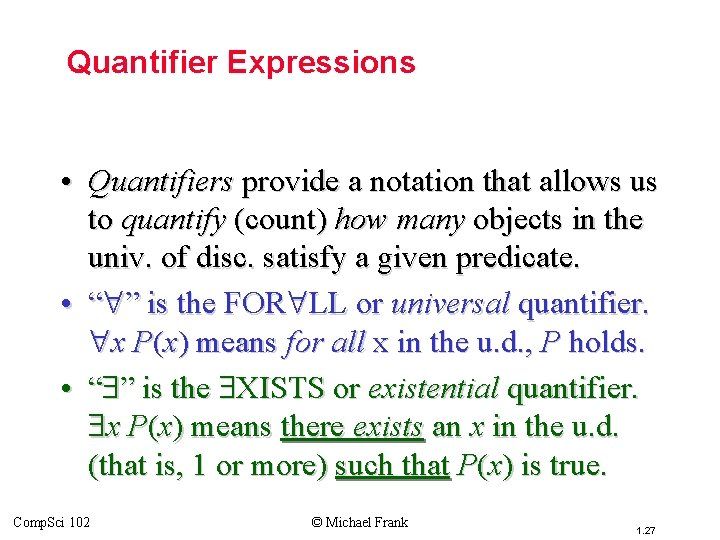Topic #3 – Predicate Logic Quantifier Expressions • Quantifiers provide a notation that allows us to quantify (count) how many objects in the univ. of disc. satisfy a given predicate. • “ ” is the FOR LL or universal quantifier. x P(x) means for all x in the u. d. , P holds. • “ ” is the XISTS or existential quantifier. x P(x) means there exists an x in the u. d. (that is, 1 or more) such that P(x) is true. Comp. Sci 102 © Michael Frank 1. 27Topic #3 – Predicate Logic The Universal Quantifier • Example: Let the u. d. of x be parking spaces at Duke. Let P(x) be the predicate “x is full. ” Then the universal quantification of P(x), x P(x), is the proposition: – “All parking spaces at Duke are full. ” – i. e. , “Every parking space at Duke is full. ” – i. e. , “For each parking space at Duke, that space is full. ” Comp. Sci 102 © Michael Frank 1. 28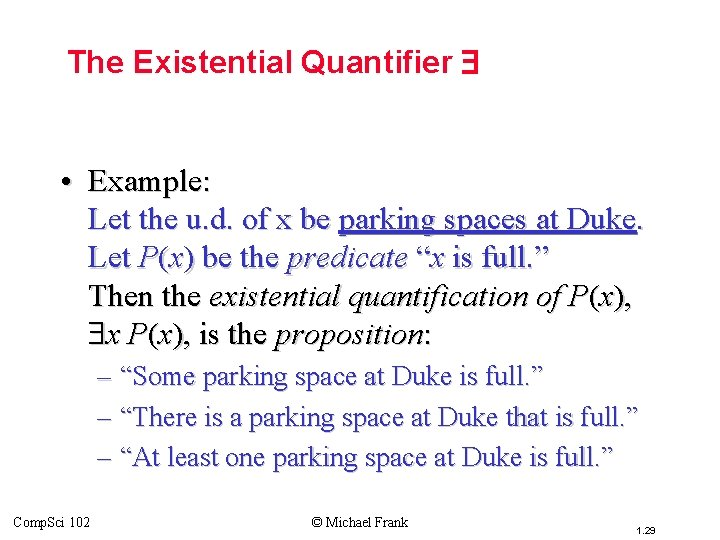Topic #3 – Predicate Logic The Existential Quantifier • Example: Let the u. d. of x be parking spaces at Duke. Let P(x) be the predicate “x is full. ” Then the existential quantification of P(x), x P(x), is the proposition: – “Some parking space at Duke is full. ” – “There is a parking space at Duke that is full. ” – “At least one parking space at Duke is full. ” Comp. Sci 102 © Michael Frank 1. 29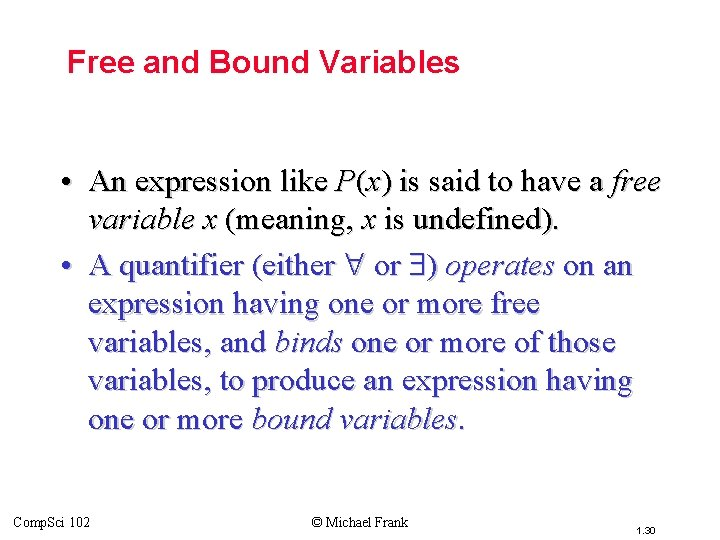Topic #3 – Predicate Logic Free and Bound Variables • An expression like P(x) is said to have a free variable x (meaning, x is undefined). • A quantifier (either or ) operates on an expression having one or more free variables, and binds one or more of those variables, to produce an expression having one or more bound variables. Comp. Sci 102 © Michael Frank 1. 30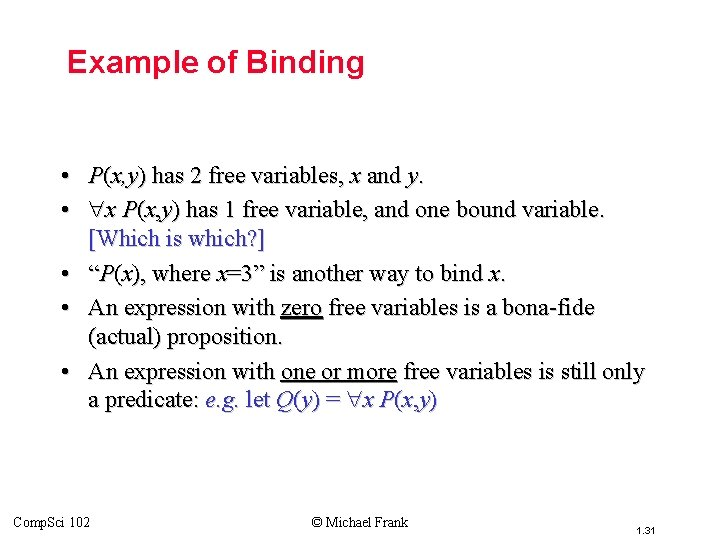Topic #3 – Predicate Logic Example of Binding • P(x, y) has 2 free variables, x and y. • x P(x, y) has 1 free variable, and one bound variable. [Which is which? ] • “P(x), where x=3” is another way to bind x. • An expression with zero free variables is a bona-fide (actual) proposition. • An expression with one or more free variables is still only a predicate: e. g. let Q(y) = x P(x, y) Comp. Sci 102 © Michael Frank 1. 31Topic #3 – Predicate Logic Nesting of Quantifiers Example: Let the u. d. of x & y be people. Let L(x, y)=“x likes y” (a predicate w. 2 f. v. ’s) Then y L(x, y) = “There is someone whom x likes. ” (A predicate w. 1 free variable, x) Then x ( y L(x, y)) = “Everyone has someone whom they like. ” (A _____ with ___ free variables. ) Comp. Sci 102 © Michael Frank 1. 32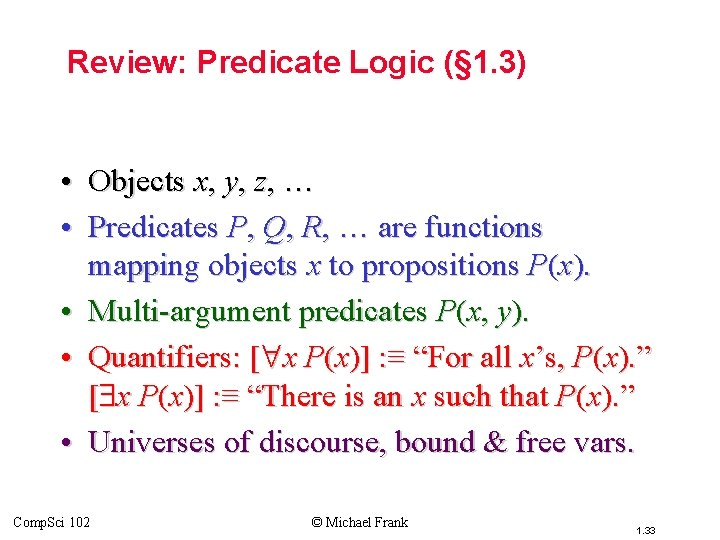Review: Predicate Logic (§ 1. 3) • Objects x, y, z, … • Predicates P, Q, R, … are functions mapping objects x to propositions P(x). • Multi-argument predicates P(x, y). • Quantifiers: [ x P(x)] : ≡ “For all x’s, P(x). ” [ x P(x)] : ≡ “There is an x such that P(x). ” • Universes of discourse, bound & free vars. Comp. Sci 102 © Michael Frank 1. 33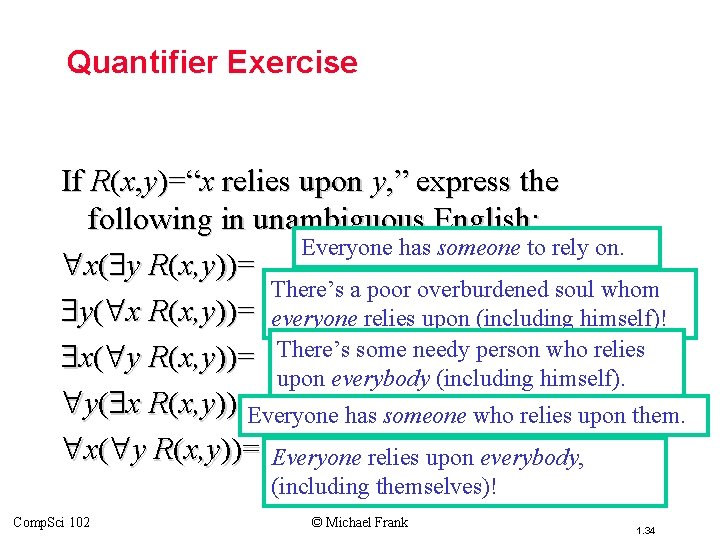Topic #3 – Predicate Logic Quantifier Exercise If R(x, y)=“x relies upon y, ” express the following in unambiguous English: Everyone has someone to rely on. x( y R(x, y))= There’s a poor overburdened soul whom y( x R(x, y))= everyone relies upon (including himself)! x( y R(x, y))= There’s some needy person who relies upon everybody (including himself). y( x R(x, y))=Everyone has someone who relies upon them. x( y R(x, y))= Everyone relies upon everybody, (including themselves)! Comp. Sci 102 © Michael Frank 1. 34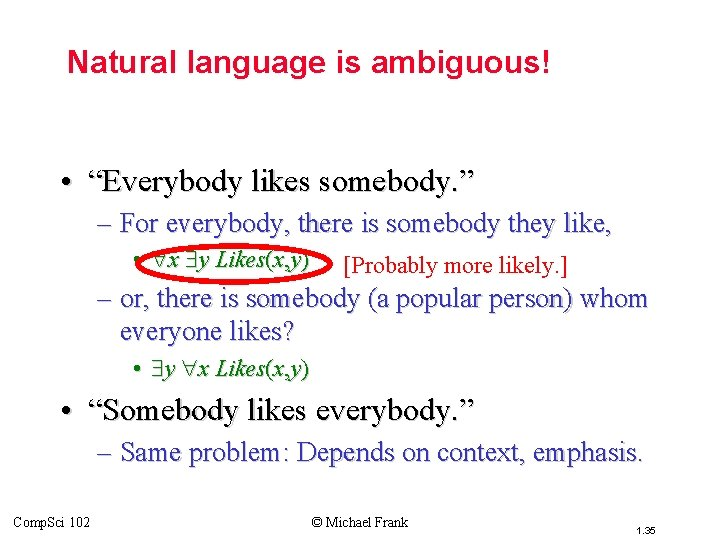Topic #3 – Predicate Logic Natural language is ambiguous! • “Everybody likes somebody. ” – For everybody, there is somebody they like, • x y Likes(x, y) [Probably more likely. ] – or, there is somebody (a popular person) whom everyone likes? • y x Likes(x, y) • “Somebody likes everybody. ” – Same problem: Depends on context, emphasis. Comp. Sci 102 © Michael Frank 1. 35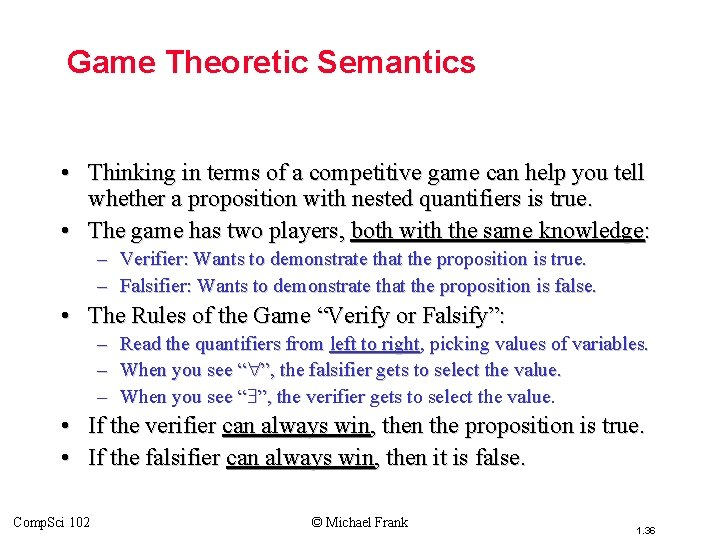Topic #3 – Predicate Logic Game Theoretic Semantics • Thinking in terms of a competitive game can help you tell whether a proposition with nested quantifiers is true. • The game has two players, both with the same knowledge: – Verifier: Wants to demonstrate that the proposition is true. – Falsifier: Wants to demonstrate that the proposition is false. • The Rules of the Game “Verify or Falsify”: – Read the quantifiers from left to right, picking values of variables. – When you see “ ”, the falsifier gets to select the value. – When you see “ ”, the verifier gets to select the value. • If the verifier can always win, then the proposition is true. • If the falsifier can always win, then it is false. Comp. Sci 102 © Michael Frank 1. 36Topic #3 – Predicate Logic Let’s Play, “Verify or Falsify!” Let B(x, y) : ≡ “x’s birthday is followed within 7 days by y’s birthday. ” Suppose I claim that among you: • Let’s play it in class. x y B(x, y) • Who wins this game? Your turn, as falsifier: • What if I switched the You pick any x → (so-and-so) quantifiers, and I y B(so-and-so, y) claimed that My turn, as verifier: y x B(x, y)? I pick any y → (such-and-such) Who wins in that case? B(so-and-so, such-and-such) Comp. Sci 102 © Michael Frank 1. 37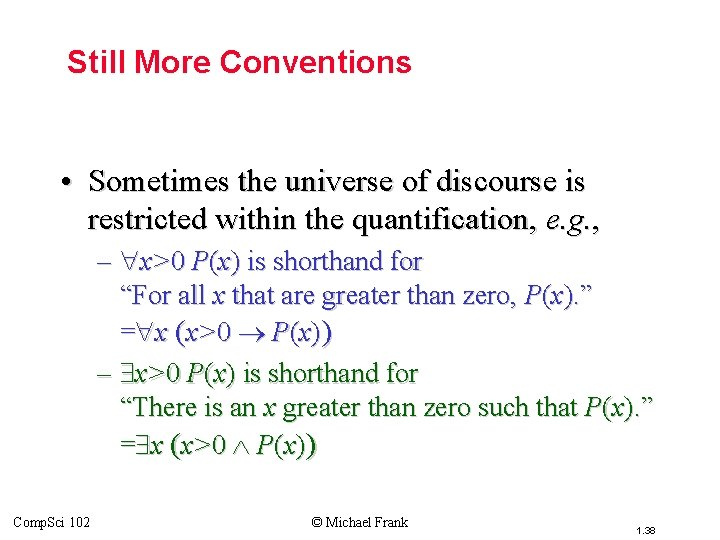Topic #3 – Predicate Logic Still More Conventions • Sometimes the universe of discourse is restricted within the quantification, e. g. , – x>0 P(x) is shorthand for “For all x that are greater than zero, P(x). ” = x (x>0 P(x)) – x>0 P(x) is shorthand for “There is an x greater than zero such that P(x). ” = x (x>0 P(x)) Comp. Sci 102 © Michael Frank 1. 38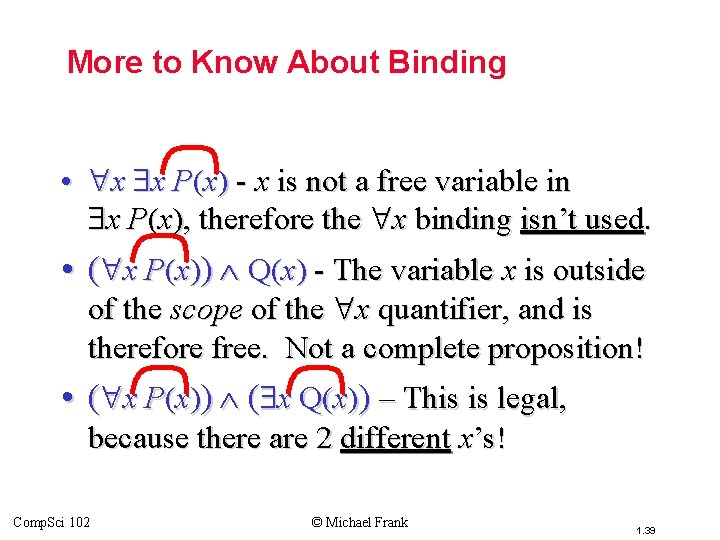Topic #3 – Predicate Logic More to Know About Binding • x x P(x) - x is not a free variable in x P(x), therefore the x binding isn’t used. • ( x P(x)) Q(x) - The variable x is outside of the scope of the x quantifier, and is therefore free. Not a complete proposition! • ( x P(x)) ( x Q(x)) – This is legal, because there are 2 different x’s! Comp. Sci 102 © Michael Frank 1. 39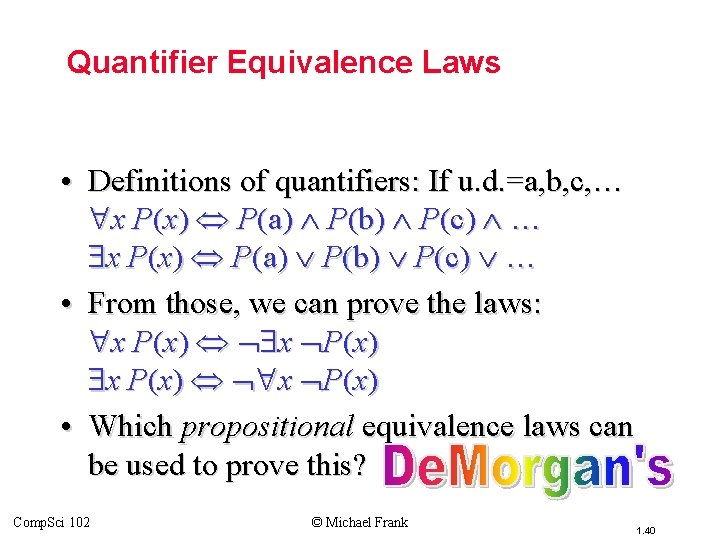Topic #3 – Predicate Logic Quantifier Equivalence Laws • Definitions of quantifiers: If u. d. =a, b, c, … x P(x) P(a) P(b) P(c) … • From those, we can prove the laws: x P(x) • Which propositional equivalence laws can be used to prove this? Comp. Sci 102 © Michael Frank 1. 40Topic #3 – Predicate Logic More Equivalence Laws • x y P(x, y) y x P(x, y) • x (P (x ) Q (x )) ( x P (x )) ( x Q (x )) • Exercise: See if you can prove these yourself. – What propositional equivalences did you use? Comp. Sci 102 © Michael Frank 1. 41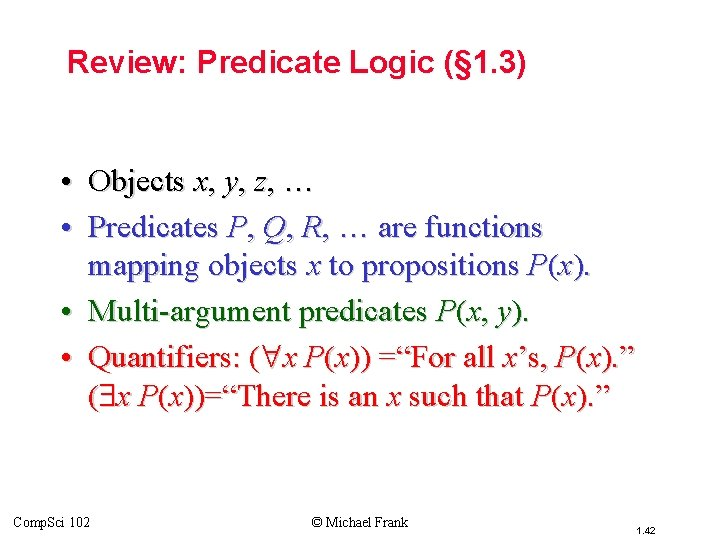Topic #3 – Predicate Logic Review: Predicate Logic (§ 1. 3) • Objects x, y, z, … • Predicates P, Q, R, … are functions mapping objects x to propositions P(x). • Multi-argument predicates P(x, y). • Quantifiers: ( x P(x)) =“For all x’s, P(x). ” ( x P(x))=“There is an x such that P(x). ” Comp. Sci 102 © Michael Frank 1. 42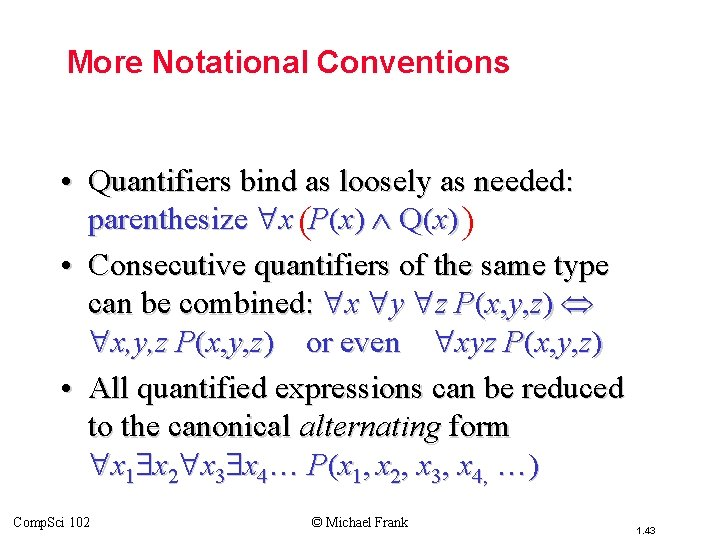Topic #3 – Predicate Logic More Notational Conventions • Quantifiers bind as loosely as needed: parenthesize x (P(x) Q(x) ) • Consecutive quantifiers of the same type can be combined: x y z P(x, y, z) x, y, z P(x, y, z) or even xyz P(x, y, z) • All quantified expressions can be reduced to the canonical alternating form x 1 x 2 x 3 x 4… P(x 1, x 2, x 3, x 4, …) Comp. Sci 102 © Michael Frank 1. 43Topic #3 – Predicate Logic Defining New Quantifiers As per their name, quantifiers can be used to express that a predicate is true of any given quantity (number) of objects. Define !x P(x) to mean “P(x) is true of exactly one x in the universe of discourse. ” !x P(x) x (P(x) y (P(y) y x)) “There is an x such that P(x), where there is no y such that P(y) and y is other than x. ” Comp. Sci 102 © Michael Frank 1. 44Topic #3 – Predicate Logic Some Number Theory Examples • Let u. d. = the natural numbers 0, 1, 2, … • “A number x is even, E(x), if and only if it is equal to 2 times some other number. ” x (E(x) ( y x=2 y)) • “A number is prime, P(x), iff it’s greater than 1 and it isn’t the product of any two non-unity numbers. ” x (P(x) (x>1 yz x=yz y 1 z 1)) Comp. Sci 102 © Michael Frank 1. 45Topic #3 – Predicate Logic Goldbach’s Conjecture (unproven) Using E(x) and P(x) from previous slide, E(x>2): P(p), P(q): p+q = x or, with more explicit notation: x [x>2 E(x)] → p q P(p) P(q) p+q = x. “Every even number greater than 2 is the sum of two primes. ” Comp. Sci 102 © Michael Frank 1. 46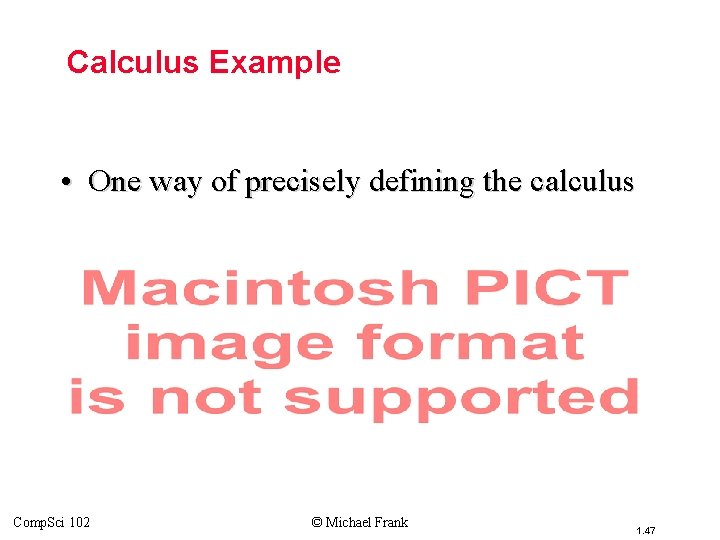Topic #3 – Predicate Logic Calculus Example • One way of precisely defining the calculus concept of a limit, using quantifiers: Comp. Sci 102 © Michael Frank 1. 47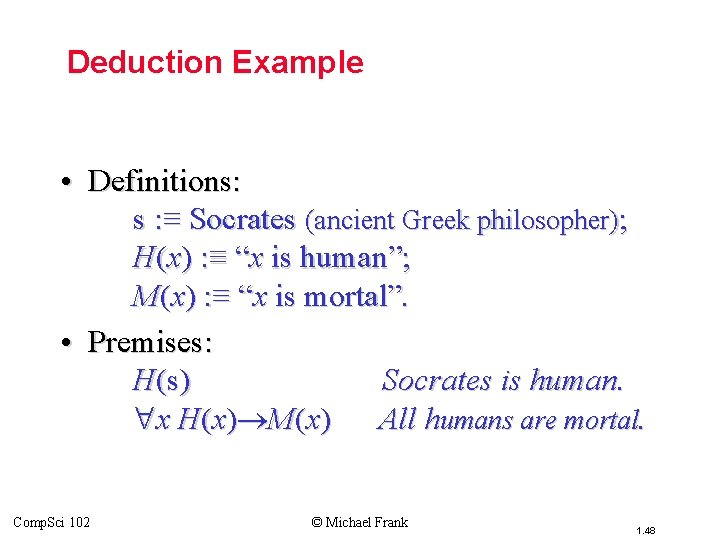Topic #3 – Predicate Logic Deduction Example • Definitions: s : ≡ Socrates (ancient Greek philosopher); H(x) : ≡ “x is human”; M(x) : ≡ “x is mortal”. • Premises: H(s) Socrates is human. x H (x ) M(x ) All humans are mortal. Comp. Sci 102 © Michael Frank 1. 48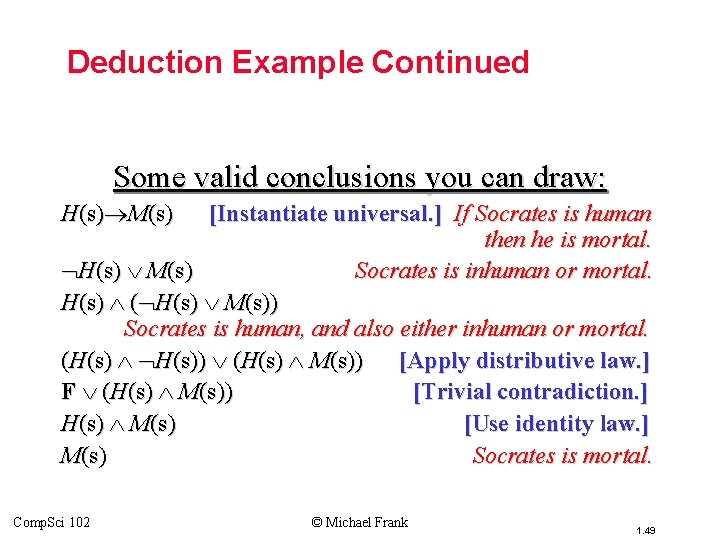Topic #3 – Predicate Logic Deduction Example Continued Some valid conclusions you can draw: H(s) M(s) [Instantiate universal. ] If Socrates is human then he is mortal. H(s) M(s) Socrates is inhuman or mortal. H(s) ( H(s) M(s)) Socrates is human, and also either inhuman or mortal. (H(s) H(s)) (H(s) M(s)) [Apply distributive law. ] F (H(s) M(s)) [Trivial contradiction. ] H(s) M(s) [Use identity law. ] M(s) Socrates is mortal. Comp. Sci 102 © Michael Frank 1. 49Topic #3 – Predicate Logic Another Example • Definitions: H(x) : ≡ “x is human”; M(x) : ≡ “x is mortal”; G(x) : ≡ “x is a god” • Premises: – x H(x) M(x) (“Humans are mortal”) and – x G(x) M(x) (“Gods are immortal”). • Show that x (H(x) G(x)) (“No human is a god. ”) Comp. Sci 102 © Michael Frank 1. 50Topic #3 – Predicate Logic The Derivation • • x H(x) M(x) and x G(x) M(x). x M(x) H(x) [Contrapositive. ] x [G(x) M(x)] [ M(x) H(x)] x G(x) H(x) [Transitivity of . ] x G(x) H(x) [Definition of . ] x (G(x) H(x)) [De. Morgan’s law. ] x G(x) H(x) [An equivalence law. ] Comp. Sci 102 © Michael Frank 1. 51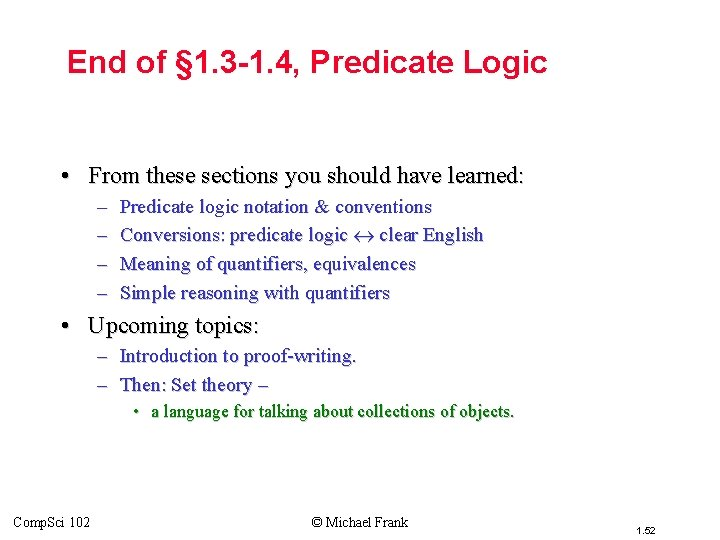Topic #3 – Predicate Logic End of § 1. 3 -1. 4, Predicate Logic • From these sections you should have learned: – – Predicate logic notation & conventions Conversions: predicate logic clear English Meaning of quantifiers, equivalences Simple reasoning with quantifiers • Upcoming topics: – Introduction to proof-writing. – Then: Set theory – • a language for talking about collections of objects. Comp. Sci 102 © Michael Frank 1. 52